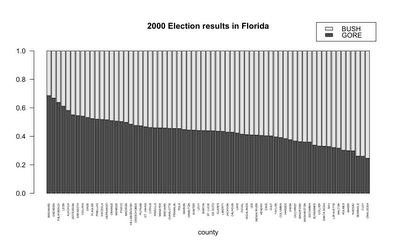# Using R for Introductory Statistics, 3.1

May 21, 2010
By

Want to share your content on R-bloggers? click here if you have a blog, or here if you don't.

#### Pairs of categorical data

The grades data.frame holds two columns of letter grades, giving pairs of categorical data, like so:

```    prev grade
1    B+    B+
2    A-    A-
3    B+    A-
...
122  B     B
```

This type of data can be summarized by the table function, which counts the occurrence of each possible pair of letter grades. But first, I was never a fan of plus-minus grading, so lets do away with that.

```> grades2 <- data.frame( prev=factor(gsub("[+]|-| ", "", as.character(grades\$prev)), levels=c('A','B','C','D','F')), grade=factor(gsub("[+]|-| ", "", as.character(grades\$grade)), levels=c('A','B','C','D','F')) )
```
```> table(grades2)
prev  A  B  C  D  F
A 22  6  3  2  0
B  4 15  5  1  3
C  3  2  9  9  7
D  0  1  4  3  1
F  1  2  4  4 11
```

You might want to compute row (1) or column (2) sums, using margin.table:

```> margin.table(table(grades2), 1)
prev
A  B  C  D  F
33 28 30  9 22
```

Of the students who got an A on the first test, what proportion also got an A on the second test? Those types of questions are answered by prop.table().

```> options(digits=1)
prev    A    B    C    D    F
A 0.67 0.18 0.09 0.06 0.00
B 0.14 0.54 0.18 0.04 0.11
C 0.10 0.07 0.30 0.30 0.23
D 0.00 0.11 0.44 0.33 0.11
F 0.05 0.09 0.18 0.18 0.50
> options(digits=4)
```

Finally, this type of data can be displayed as a stacked barplot.

```m <- t(as.matrix(florida[,2:3]))
m.prop <- prop.table(m, margin=2)
colnames(m.prop) <- florida\$County

# fool around with margins and set style of axis labels
# mar=c(bottom, left, top, right)
# las=2 => always perpendicular to the axis
old = par(mar=c(6,4,6,2)+0.1, las=2)

# cex.names => "character expansion" of bar labels
# args.legend => position the legend out of the plot area
barplot(m.prop[,order(m.prop[2,])], legend.text=T, cex.names=0.40, args.legend=list(x=82,y=1.2), main="2000 Election results in Florida", sub='county')

# reset old parameters
par(old)
```R-bloggers.com offers daily e-mail updates about R news and tutorials about learning R and many other topics. Click here if you're looking to post or find an R/data-science job.
Want to share your content on R-bloggers? click here if you have a blog, or here if you don't.

Tags: , ,